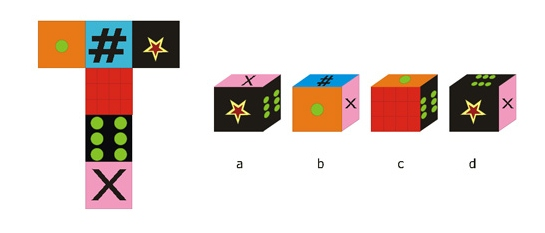## CSCI 580: 3D Graphics and Rendering

### Spr' 2012 - Final Exam

#### 5/2/12, 6:30-8:00 PM

``

Name: ____________________________________

Student ID: ______________________________

1   1
2   1
3   1
4   1
5   1
6   1
7   1
8   1
9   1
10   1
11   1
12   1
13   1
14   1
15   1
16   1
17   1
18   1
19   1
20   1
Bonus   2
Total   22

THINK, DO WELL, ENJOY!
[not necessarily in that order!]

#### Question 1 [1 point]

What is (makes for) 3D computer graphics? [the answer is NOT 'stereo'!]

#### Question 2 [1 point]

What is computer graphics 'rendering' ?

#### Question 3 [0.5 + 0.5 = 1 point]

In CG, what is a 'model'? Also, mention another area/field where the word 'model' is used.

#### Question 4 [0.5 + 0.5 = 1 point]

In CG rendering (or digital images in general), what is 'resolution'? Also, what is another area/field where 'resolution' is used?

#### Question 5 [1 point]

What is stored in a 'framebuffer'?

#### Question 6 [0.5+0.5 = 1 point]

What do 'LEE' and 'DDA' stand for, in the context of scan conversion?

#### Question 7 [0.5+0.5 = 1 point]

Mention any two hidden-surface algorithms.

#### Question 8 [0.5+0.5 = 1 point]

What are a couple of properties related to translation through 3D space?

#### Question 9 [0.2*5 = 1 point]

What are the five distinct co-ordinate 'spaces' used in the classic rendering pipeline?

#### Question 10 [1 point]

What are a couple of differences between local and global illumination?

#### Question 11 [1 point]

Name the three components (parts) that make up the classic lighting equation.

#### Question 12 [1 point]

When it comes to parameter interpolation (eg. of uv texture coords) during scan conversion-based rendering, what does "perspective correction" mean?

#### Question 13 [1 point]

Explain BRIEFLY how texture mapping works (ie. how an image ("texture map") gets on to a 3D rendered surface).

#### Question 14 [0.5+0.5 = 1 point]

How does bump mapping differ from displacement mapping? Why even have these two different techniques (why not always just use one of them)?

#### Question 15 [0.5+0.5 = 1 point]

In addition to being used for texturing, maps have several other uses as well - name two.

#### Question 16 [1 point]

State the Nyquist sampling theorem in simple words, along with a (simple) diagram.

#### Question 18 [1 point]

How does the NPR technique of toon-shading work? Explain, using a simple figure.

#### Question 19 [0.5+0.5 = 1 point]

What is a 'lightfield'? Name one practical (commercial) device that can record (capture) it.

#### Question 20 [0.25*4 = 1 point]

Name any four commercially available renderers.

#### Bonus (non-CG) [2 points]

Look at the flattened cube on the left, in the figure below. Which of the cubes on the right (a or b or c or d) could have produced the flattened version?